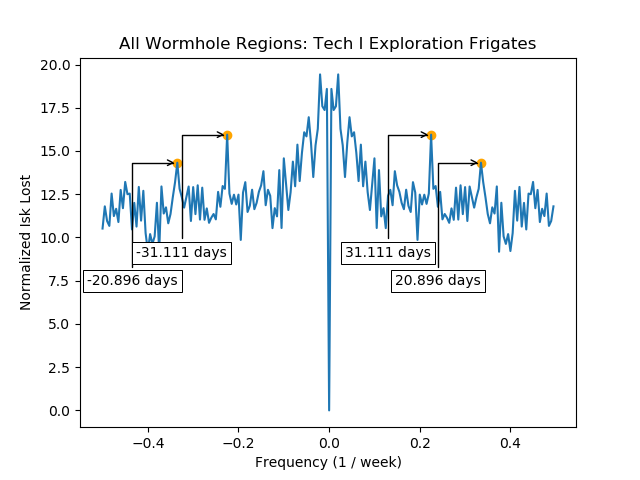# Short story

If I count all wormhole regions, loot fairy slightly prefers to come every month. In some wormhole regions, loot fairy prefers to come every X days. But X is generally different for different regions.

Average of Regional Averages
|The spectrum shows periodic fluctuations in the amount of Isk lost by T1 exploration frigates.The time series shows the normalized Isk lost over time.
Regional Averages

Big table of graphs for each wormhole region
Region Spectrum Time Series
A-R00001 spectrum_A-R00001 time_series_A-R00001
A-R00002 spectrum_A-R00002 time_series_A-R00002
A-R00003 spectrum_A-R00003 time_series_A-R00003
B-R00004 spectrum_B-R00004 time_series_B-R00004
B-R00005 spectrum_B-R00005 time_series_B-R00005
B-R00006 spectrum_B-R00006 time_series_B-R00006
B-R00007 spectrum_B-R00007 time_series_B-R00007
B-R00008 spectrum_B-R00008 time_series_B-R00008
C-R00009 spectrum_C-R00009 time_series_C-R00009
C-R00010 spectrum_C-R00010 time_series_C-R00010
C-R00011 spectrum_C-R00011 time_series_C-R00011
C-R00012 spectrum_C-R00012 time_series_C-R00012
C-R00013 spectrum_C-R00013 time_series_C-R00013
C-R00014 spectrum_C-R00014 time_series_C-R00014
C-R00015 spectrum_C-R00015 time_series_C-R00015
D-R00016 spectrum_D-R00016 time_series_D-R00016
D-R00017 spectrum_D-R00017 time_series_D-R00017
D-R00018 spectrum_D-R00018 time_series_D-R00018
D-R00019 spectrum_D-R00019 time_series_D-R00019
D-R00020 spectrum_D-R00020 time_series_D-R00020
D-R00021 spectrum_D-R00021 time_series_D-R00021
D-R00022 spectrum_D-R00022 time_series_D-R00022
D-R00023 spectrum_D-R00023 time_series_D-R00023
E-R00024 spectrum_E-R00024 time_series_E-R00024
E-R00025 spectrum_E-R00025 time_series_E-R00025
E-R00026 spectrum_E-R00026 time_series_E-R00026
E-R00027 spectrum_E-R00027 time_series_E-R00027
E-R00028 spectrum_E-R00028 time_series_E-R00028
E-R00029 spectrum_E-R00029 time_series_E-R00029
F-R00030 spectrum_F-R00030 time_series_F-R00030
G-R00031 spectrum_G-R00031 time_series_G-R00031
H-R00032 spectrum_H-R00032 time_series_H-R00032
K-R00033 spectrum_K-R00033 time_series_K-R00033

# Details

• For every week, I calculate the normalized Isk lost in a region with two steps:
1. I calculate (raw isk lost) = (Isk lost in this worm hole region) / [(#Ship killed in this region) * (Isk lost all the worm hole regions) ]
This should remove the effect of inflation and account for the regional differences of the amount of ships killed.
2. Standardize the (raw isk lost) over all weeks. I subtract the average over time from all the (raw isk lost). Then I divide the result by the standard deviation.
This would make all the spectra for all regions have similar magnitudes.
• The average of regional average is: average of normalized Isk lost over all regions.
Notice that this give all regions equal importance. The regions don’t have the same number of systems.
• The data is from the monthly economic reports and a kill dump for March, 2016.
• I group kills every 7 days to remove the strong weekly cycle.
2 Likes

Have you sacrificed a ship at the sun to appease Bob?

1 Like

interdasting

And what is the conclusion?

“Does Loot Fairy Come Periodically in Wormholes?”

You gotta ask Bob about that … ( ͡° ͜ʖ ͡° )

This topic was automatically closed 90 days after the last reply. New replies are no longer allowed.